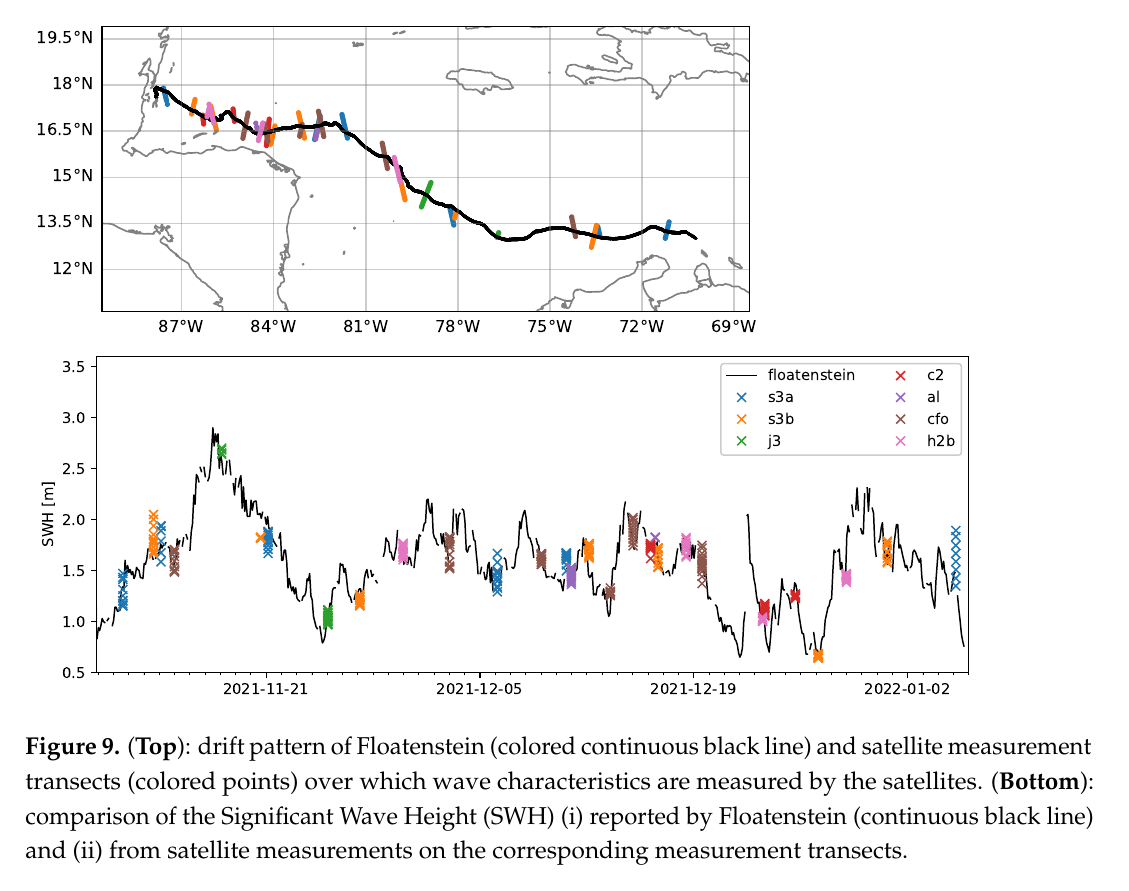# One Ocean Expedition

The following examples are drawn from work where wavy was used related to the One Ocean Expedition.

## 1. Collocating satellite tracks with drifter

In Rabault et al. (2022) we compared a drifter (floatenstein) that can measure waves to satellite altimetry. To do something similar you need to obtain the following drifter time series as lists: - time as datetime objects - longitudes - latitudes

Those need to be formulated in a dictionary such that wavy can parse it.

```>>> poi_dict = {'datetime':dt,'longitude':lons,'latitude':lats}
```

Now load the satellite class from wavy and hit play:

```>>> from wavy.satmod import satellite_class as sc
>>> sco = sc(twin=30,distlim=50,mission='j3',region='global',poi=poi_dict)
```

After you can save the results as pickle or netcdf and make a figure as in Rabault et al. (2022).## 2. Collocating model output with drifter

In a different experiment we compare Hs from a wave sensor onboard Statsraad Lemkuhl with the ecmwf wave model ecwam. Again we need from the instrument: - time as datetime objects - longitudes - latitudes - observations - and some name (nID)

Those need to be formulated in a dictionary such that wavy can parse it.

```>>> poi_dict = {'time':dt,'longitude':lons,'latitude':lats,'obs':obs,'nID':'OneOcean'}
```

Import wavy’s collocation class add some collocation specifications and hit play:

```>>> from wavy.collocmod import collocation_class as cc
>>> cco = cc(poi=poi_dict,model='ecwam_Hs',twin=30,date_incr=1,varalias='Hs',distlim=30)
```

Note

The observations may consist of missing values of the same length as the other parameters in case only a trajectory is of interest and not actual values from a drifter.